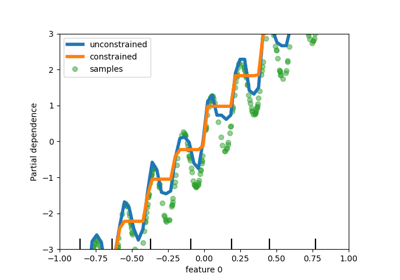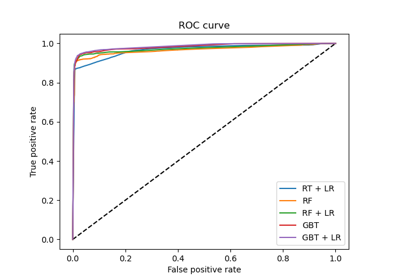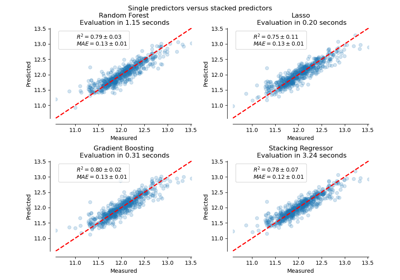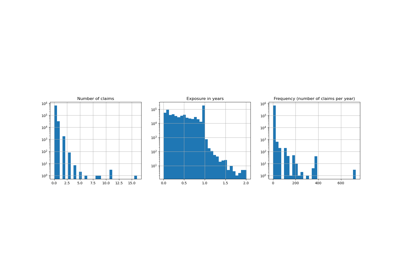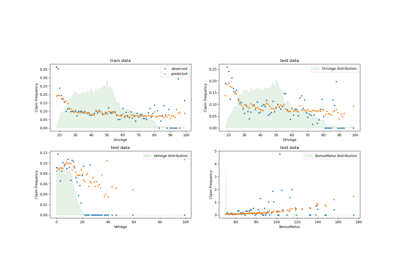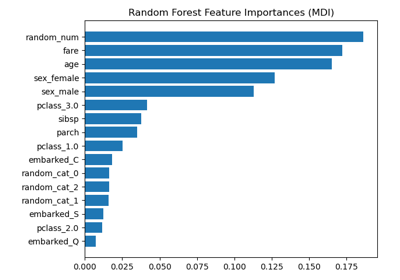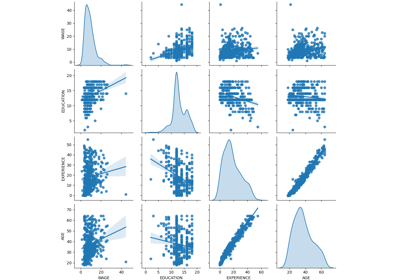# sklearn.preprocessing.OneHotEncoder¶

class sklearn.preprocessing.OneHotEncoder(*, categories='auto', drop=None, sparse=True, dtype=<class 'numpy.float64'>, handle_unknown='error')[source]

Encode categorical features as a one-hot numeric array.

The input to this transformer should be an array-like of integers or strings, denoting the values taken on by categorical (discrete) features. The features are encoded using a one-hot (aka ‘one-of-K’ or ‘dummy’) encoding scheme. This creates a binary column for each category and returns a sparse matrix or dense array (depending on the sparse parameter)

By default, the encoder derives the categories based on the unique values in each feature. Alternatively, you can also specify the categories manually.

This encoding is needed for feeding categorical data to many scikit-learn estimators, notably linear models and SVMs with the standard kernels.

Note: a one-hot encoding of y labels should use a LabelBinarizer instead.

Read more in the User Guide.

Changed in version 0.20.

Parameters
categories‘auto’ or a list of array-like, default=’auto’

Categories (unique values) per feature:

• ‘auto’ : Determine categories automatically from the training data.

• list : categories[i] holds the categories expected in the ith column. The passed categories should not mix strings and numeric values within a single feature, and should be sorted in case of numeric values.

The used categories can be found in the categories_ attribute.

New in version 0.20.

drop{‘first’, ‘if_binary’} or a array-like of shape (n_features,), default=None

Specifies a methodology to use to drop one of the categories per feature. This is useful in situations where perfectly collinear features cause problems, such as when feeding the resulting data into a neural network or an unregularized regression.

However, dropping one category breaks the symmetry of the original representation and can therefore induce a bias in downstream models, for instance for penalized linear classification or regression models.

• None : retain all features (the default).

• ‘first’ : drop the first category in each feature. If only one category is present, the feature will be dropped entirely.

• ‘if_binary’ : drop the first category in each feature with two categories. Features with 1 or more than 2 categories are left intact.

• array : drop[i] is the category in feature X[:, i] that should be dropped.

sparsebool, default=True

Will return sparse matrix if set True else will return an array.

dtypenumber type, default=np.float

Desired dtype of output.

handle_unknown{‘error’, ‘ignore’}, default=’error’

Whether to raise an error or ignore if an unknown categorical feature is present during transform (default is to raise). When this parameter is set to ‘ignore’ and an unknown category is encountered during transform, the resulting one-hot encoded columns for this feature will be all zeros. In the inverse transform, an unknown category will be denoted as None.

Attributes
categories_list of arrays

The categories of each feature determined during fitting (in order of the features in X and corresponding with the output of transform). This includes the category specified in drop (if any).

drop_idx_array of shape (n_features,)
• drop_idx_[i] is the index in categories_[i] of the category to be dropped for each feature.

• drop_idx_[i] = None if no category is to be dropped from the feature with index i, e.g. when drop='if_binary' and the feature isn’t binary.

• drop_idx_ = None if all the transformed features will be retained.

sklearn.preprocessing.OrdinalEncoder

Performs an ordinal (integer) encoding of the categorical features.

sklearn.feature_extraction.DictVectorizer

Performs a one-hot encoding of dictionary items (also handles string-valued features).

sklearn.feature_extraction.FeatureHasher

Performs an approximate one-hot encoding of dictionary items or strings.

sklearn.preprocessing.LabelBinarizer

Binarizes labels in a one-vs-all fashion.

sklearn.preprocessing.MultiLabelBinarizer

Transforms between iterable of iterables and a multilabel format, e.g. a (samples x classes) binary matrix indicating the presence of a class label.

Examples

Given a dataset with two features, we let the encoder find the unique values per feature and transform the data to a binary one-hot encoding.

>>> from sklearn.preprocessing import OneHotEncoder


One can discard categories not seen during fit:

>>> enc = OneHotEncoder(handle_unknown='ignore')
>>> X = [['Male', 1], ['Female', 3], ['Female', 2]]
>>> enc.fit(X)
OneHotEncoder(handle_unknown='ignore')
>>> enc.categories_
[array(['Female', 'Male'], dtype=object), array([1, 2, 3], dtype=object)]
>>> enc.transform([['Female', 1], ['Male', 4]]).toarray()
array([[1., 0., 1., 0., 0.],
[0., 1., 0., 0., 0.]])
>>> enc.inverse_transform([[0, 1, 1, 0, 0], [0, 0, 0, 1, 0]])
array([['Male', 1],
[None, 2]], dtype=object)
>>> enc.get_feature_names(['gender', 'group'])
array(['gender_Female', 'gender_Male', 'group_1', 'group_2', 'group_3'],
dtype=object)


One can always drop the first column for each feature:

>>> drop_enc = OneHotEncoder(drop='first').fit(X)
>>> drop_enc.categories_
[array(['Female', 'Male'], dtype=object), array([1, 2, 3], dtype=object)]
>>> drop_enc.transform([['Female', 1], ['Male', 2]]).toarray()
array([[0., 0., 0.],
[1., 1., 0.]])


Or drop a column for feature only having 2 categories:

>>> drop_binary_enc = OneHotEncoder(drop='if_binary').fit(X)
>>> drop_binary_enc.transform([['Female', 1], ['Male', 2]]).toarray()
array([[0., 1., 0., 0.],
[1., 0., 1., 0.]])


Methods

 fit(X[, y]) Fit OneHotEncoder to X. fit_transform(X[, y]) Fit OneHotEncoder to X, then transform X. get_feature_names([input_features]) Return feature names for output features. get_params([deep]) Get parameters for this estimator. Convert the data back to the original representation. set_params(**params) Set the parameters of this estimator. Transform X using one-hot encoding.
__init__(*, categories='auto', drop=None, sparse=True, dtype=<class 'numpy.float64'>, handle_unknown='error')[source]

Initialize self. See help(type(self)) for accurate signature.

fit(X, y=None)[source]

Fit OneHotEncoder to X.

Parameters
Xarray-like, shape [n_samples, n_features]

The data to determine the categories of each feature.

yNone

Ignored. This parameter exists only for compatibility with sklearn.pipeline.Pipeline.

Returns
self
fit_transform(X, y=None)[source]

Fit OneHotEncoder to X, then transform X.

Equivalent to fit(X).transform(X) but more convenient.

Parameters
Xarray-like, shape [n_samples, n_features]

The data to encode.

yNone

Ignored. This parameter exists only for compatibility with sklearn.pipeline.Pipeline.

Returns
X_outsparse matrix if sparse=True else a 2-d array

Transformed input.

get_feature_names(input_features=None)[source]

Return feature names for output features.

Parameters
input_featureslist of str of shape (n_features,)

String names for input features if available. By default, “x0”, “x1”, … “xn_features” is used.

Returns
output_feature_namesndarray of shape (n_output_features,)

Array of feature names.

get_params(deep=True)[source]

Get parameters for this estimator.

Parameters
deepbool, default=True

If True, will return the parameters for this estimator and contained subobjects that are estimators.

Returns
paramsmapping of string to any

Parameter names mapped to their values.

inverse_transform(X)[source]

Convert the data back to the original representation.

In case unknown categories are encountered (all zeros in the one-hot encoding), None is used to represent this category.

Parameters
Xarray-like or sparse matrix, shape [n_samples, n_encoded_features]

The transformed data.

Returns
X_trarray-like, shape [n_samples, n_features]

Inverse transformed array.

set_params(**params)[source]

Set the parameters of this estimator.

The method works on simple estimators as well as on nested objects (such as pipelines). The latter have parameters of the form <component>__<parameter> so that it’s possible to update each component of a nested object.

Parameters
**paramsdict

Estimator parameters.

Returns
selfobject

Estimator instance.

transform(X)[source]

Transform X using one-hot encoding.

Parameters
Xarray-like, shape [n_samples, n_features]

The data to encode.

Returns
X_outsparse matrix if sparse=True else a 2-d array

Transformed input.

## Examples using sklearn.preprocessing.OneHotEncoder¶